Electron. J. Diff. Equ., Vol. 2013 (2013), No. 195, pp. 1-8.

### Limit cycles for discontinuous generalized Lienard polynomial differential equations Jaume Llibre, Ana Cristina Mereu

Abstract:
We divide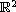into sectors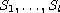, with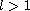even, and define a discontinuous differential system such that in each sector, we have a smooth generalized Lienard polynomial differential equation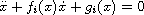,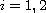alternatively, where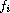and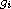are polynomials of degree n-1 and m respectively. Then we apply the averaging theory for first-order discontinuous differential systems to show that for anyandthere are non-smooth Lienard polynomial equations having at least max{n,m} limit cycles. Note that this number is independent of the number of sectors.

Submitted May 7, 2013. Published September 3, 2013.
Math Subject Classifications: 34C29, 34C25, 47H11.
Key Words: Limit cycles; non-smooth Lienard systems; averaging theory.

Show me the PDF file (221 KB), TEX file, and other files for this article.Jaume Llibre Departament de Matematiques Universitat Autonoma de Barcelona 08193 Bellaterra, Barcelona, Catalonia, Spain email: jllibre@mat.uab.cat Ana Cristina Mereu Department of Physics, Chemistry and Mathematics UFSCar 18052-780, Sorocaba, SP, Brazil email: anamereu@ufscar.br